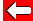# Averages: Median

The median is another useful type of average. It can be tricky to work out, as it is the number found in the middle of the set when they are listed in numerical order!

e.g. The median of {1, 2, 4, 4, 5, 8, 9} is 4 as it is the 4th number in a set of 7, so is exactly in the middle.

What about a set of numbers with an even total?
e.g. What is the median of {1, 2, 4, 5, 7, 9, 12, 20}?

There are two numbers in the middle: 5 and 7. Neither of these can be the median. We choose instead their mean average and settle for that:

The mean of the two middle numbers is (5+7) ÷ 2 = 6.Go back a pageMaths MenuGo to next page